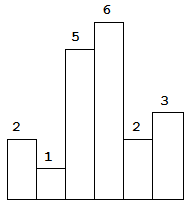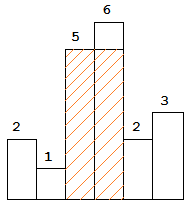## 柱状图中最大矩形1. 若当前元素大于等于栈顶元素，加入栈
2. 若当前元素小，则弹出栈顶元素，继续比较，直到它也入栈
stack<int> st;
for(int i = 0; i < nums.size(); i++)
{
while(!st.empty() && st.top() > nums[i])
{
st.pop();
}
st.push(nums[i]);
}

1. 栈内的元素是递增的
2. 当元素出栈时，说明这个新元素是出栈元素向后找第一个比其小的元素
3. 当元素出栈后，说明新栈顶元素是出栈元素向前找第一个比其小的元素1. 矩形框为弹出元素的高
2. 左边界为 弹出的栈顶元素下标，右边界为 i-1
3. 面积为max(ans, (right - left + 1) * heights[cur])
class Solution {
public:
int largestRectangleArea(vector<int>& heights) {
int ans = 0;
stack<int> st;
heights.insert(heights.begin(), 0);//在向量最前面插入0
heights.push_back(0);//在向量最后面插入0
for (int i = 0; i < heights.size(); i++)
{//st.back()存的是栈顶元素的height[]数组下标，heights[st.back()]为单调递增栈的栈顶元素
while (!st.empty() && heights[st.top()] > heights[i])
{//栈非空且当前元素小于栈顶元素，则弹出栈顶元素，并且计算面积
int cur = st.top();
st.pop();//弹出栈
int left = st.top() + 1;//左边界为 新的栈顶元素下标+1
int right = i - 1;//右边界为 i-1
ans = max(ans, (right - left + 1) * heights[cur]);
}
st.push(i);
}
return ans;
}
};

## 逛街

##### 输入描述:
输入第一行将包含一个数字n，代表楼的栋数，接下来的一行将包含n个数字wi(1<=i<=n)，代表每一栋楼的高度。
1<=n<=100000;
1<=wi<=100000; 

输出一行，包含空格分割的n个数字vi，分别代表小Q在第i栋楼时能看到的楼的数量。

6
5 3 8 3 2 5

3 3 5 4 4 4

a[i] 表示以x[i]为终点的单调递减栈的长度大小：

i从0~n-1的顺序遍历：当扫描的元素x[i]大于栈顶元素的时候，弹出栈顶元素直到有比x[i]大的元素才入栈，此时栈中就是以x[i]为终点的单调递减栈；

j从后往前的顺序（ n-1 ~ 0 ）遍历：当扫描的元素x[j]]大于栈顶元素的时候，弹出栈顶元素直到有比x[j]大的元素才入栈，此时栈中就是以x[j]为起点的单调递增栈；

#include <iostream>
#include <vector>
#include <stack>
#include <algorithm>
using namespace std;
vector<int> a, b;
stack<int> st1, st2;
int main() {
int n, x;
cin >> n;
int ans = 0;
for (int i = 0; i < n; i++) cin >> x[i];
for (int i = 0, j = n - 1; i < n, j >= 0; i++, j--) {
a.push_back(st1.size());
b.push_back(st2.size());
while (!st1.empty() && st1.top() <= x[i]) st1.pop();
while (!st2.empty() && st2.top() <= x[j]) st2.pop();
st1.push(x[i]);
st2.push(x[j]);
}
reverse(b.begin(), b.end());//由于b是从n-1开始往前计算的，所以需要翻转
for (int i = 0; i < n; i++) cout << b[i] + a[i] + 1<< " ";
return 0;
}

## 739 每日温度

• 如果栈为空，则加入当前扫描的温度
• 如果栈不为空：

• 如果扫描的温度大于栈顶的温度，说明当前扫描的温度是栈顶温度之后第一个大于它的温度，计算他们的日期之差，存入到ans[previousIndex]中；
• 如果扫描的温度小于等于栈顶的温度，说明当前的温度太低，直接入栈
class Solution {
public:
vector<int> dailyTemperatures(vector<int>& T) {
int n = T.size();
vector<int> ans(n);
stack<int> s;
for(int i = 0;i < n;++i){
while(!s.empty() && T[i] > T[s.top()]){
int previousIndex = s.top();
ans[previousIndex] = i - previousIndex;
s.pop();
}
s.push(i);
}
return ans;
}
};

## 901 股票的价格跨度

S.next(100) 被调用并返回 1，
S.next(80) 被调用并返回 1，
S.next(60) 被调用并返回 1，
S.next(70) 被调用并返回 2，
S.next(60) 被调用并返回 1，
S.next(75) 被调用并返回 4，
S.next(85) 被调用并返回 6。

(包括今天的价格 75) 小于或等于今天的价格。

class StockSpanner {
private:
stack<int> weight;
stack<int> stock;
public:
StockSpanner() {
}

int next(int price) {
int ans = 1;
while(!weight.empty()  && !stock.empty() && price >= stock.top() ){
ans += weight.top();
weight.pop();
stock.pop();
}
weight.push(ans);
stock.push(price);
return ans;
}
};

/**
* Your StockSpanner object will be instantiated and called as such:
* StockSpanner* obj = new StockSpanner();
* int param_1 = obj->next(price);
*/